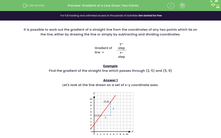# Find the Gradient of a Line Given Two Points

In this worksheet, students will calculate the gradient of a line which passes through two given points.Key stage:  KS 3

Curriculum topic:   Algebra

Curriculum subtopic:   Solve Linear Equations (One Variable)

Popular topics:   Algebra worksheets

Difficulty level:#### Worksheet Overview

It is possible to work out the gradient of a straight line from the coordinates of any two points which lie on the line, either by drawing the line or simply by subtracting and dividing coordinates.

The rule to remember is:

The gradient of a line = y-step ÷ x-step

Example

Find the gradient of the straight line that passes through (2, 5) and (5, 9)

Let's look at the line drawn on a set of x-y coordinate axes.The vertical part of the step (y-step) = 4

The horizontal part of the step (x-step) = 3

The gradient of the line = y=step ÷ x-step = 4 ÷ 3 = 4/3

It is possible to work out the gradient without drawing the line.

Look at the coordinates of the two points (2, 5) and (59).

We can get the y-step by subtracting the y-coordinates.

y-step =  9 - 5 = 4

In the same order, we get the x-step by subtracting the x-coordinates:

x-step =  5 - 2 = 3

The gradient of the line = y-step ÷ x-step = 4 ÷ 3 = 4/3

NB: We could have worked out 5 - 9 = -4 and 2 - 5 = -3 but -4 ÷ -3 = 4 ÷ 3

Want a bit more help with this before you begin? Why not watch this short video?

### What is EdPlace?

We're your National Curriculum aligned online education content provider helping each child succeed in English, maths and science from year 1 to GCSE. With an EdPlace account you’ll be able to track and measure progress, helping each child achieve their best. We build confidence and attainment by personalising each child’s learning at a level that suits them.

Get started